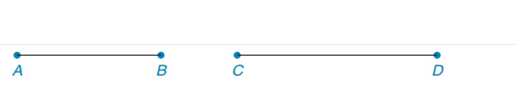Chapter 7.1, Problem 37E### Elementary Geometry for College St...

6th Edition
Daniel C. Alexander + 1 other
ISBN: 9781285195698

#### Solutions

Chapter
Section### Elementary Geometry for College St...

6th Edition
Daniel C. Alexander + 1 other
ISBN: 9781285195698
Textbook Problem
1 views

# In Exercises 37 to 40, refer to the line segments shown.Construct an isosceles right triangle that has hypotenuse A B - .To determine

To construct:

The isosceles triangle that has hypotenuse AB¯.

Explanation

Procedure:

An angle inscribed in a semicircle is a right angle triangle.

Construct a circle whose diameter is the midpoint M of the hypotenuse and whose radius equals one-half of the length of the hypotenuse.

1. Construct a line segment AB¯.

2. Locate the midpoint M.

3. Draw a line MC perpendicular to the line AB¯.

4

### Still sussing out bartleby?

Check out a sample textbook solution.

See a sample solution

#### The Solution to Your Study Problems

Bartleby provides explanations to thousands of textbook problems written by our experts, many with advanced degrees!

Get Started

#### In Exercises 5-8, solve for x. x2+7x=0

Calculus: An Applied Approach (MindTap Course List)

#### In Exercise 724, Compute the indicated products. 3

Finite Mathematics for the Managerial, Life, and Social Sciences

#### Using for |x| < 1 and differentiation, find a power series for .

Study Guide for Stewart's Single Variable Calculus: Early Transcendentals, 8th